# M6-S2: The Motor Effect and Forces Between a Pair of Straight Conductors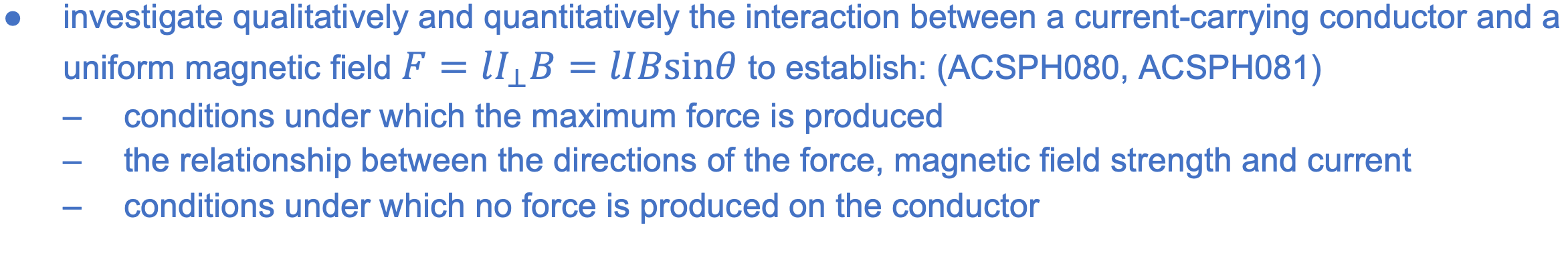### What is the Motor Effect?

• Recall that a charged particle experiences a force when an external magnetic field is applied. A current in a conductor experiences a force via the same mechanism as a current is fundamentally moving electrons.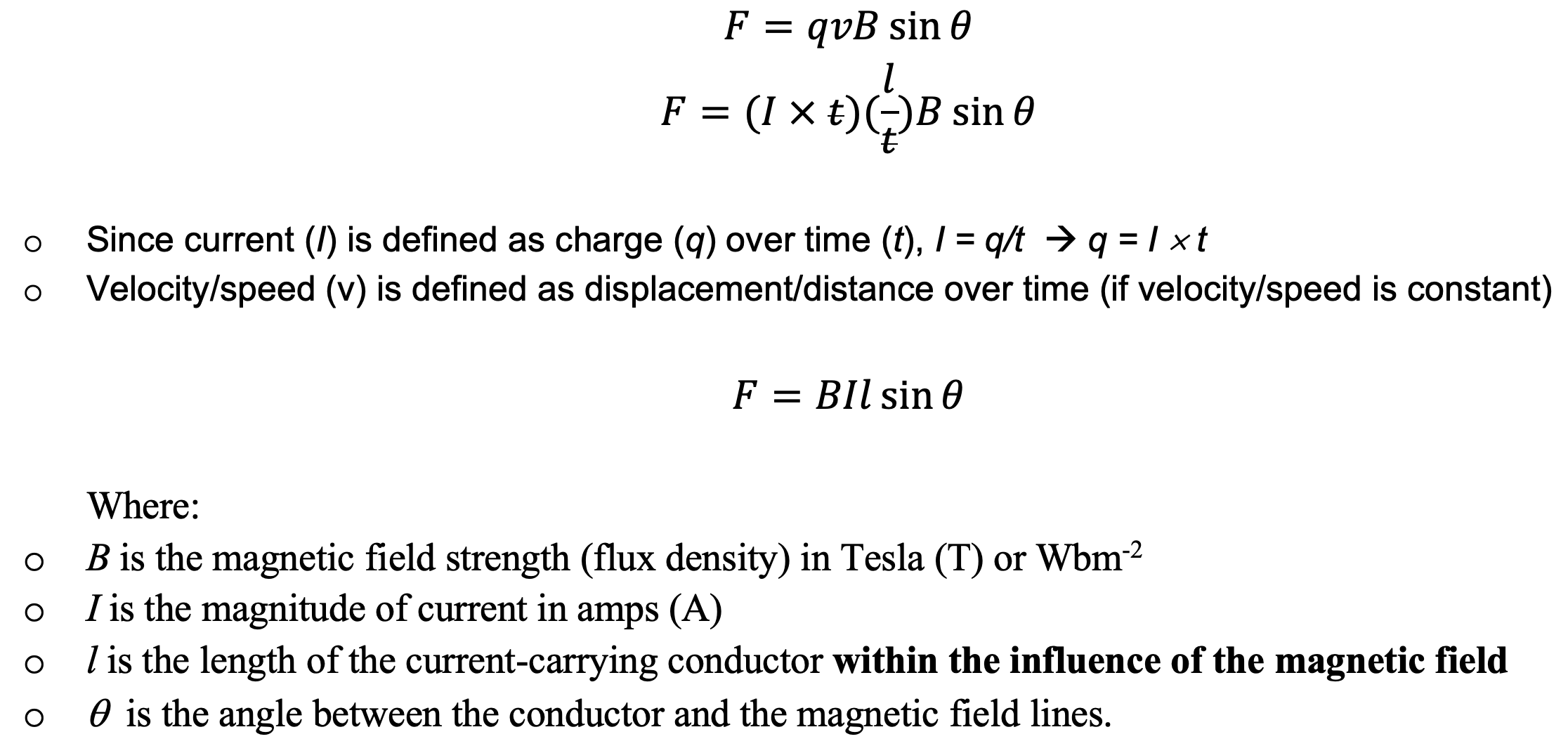• Motor effect: a current-carrying conductor experiences a force in an external magnetic field.
• Magnitude of force is directly proportional to magnetic field strength, current, length of conductor and angle between the conductor and magnetic field lines.
• Direction of force is determined by right-hand palm rule where
• Thumb points in the direction of conventional current
• Four fingers point in the direction of magnetic field lines
• Palm faces the direction of force generated by the motor effect
• Maximum force occurs when the conductor is perpendicular (q = 90º) to the magnetic field lines
• Zero force (minimum) occurs when the conductor is parallel (q = 0º) to the magnetic field lines

Investigation of the Motor Effect

• A single loop of wire is placed within a horse-show magnet and connected to an external power source (e.g. battery box)
• Make sure you know how to replicate this diagram on paper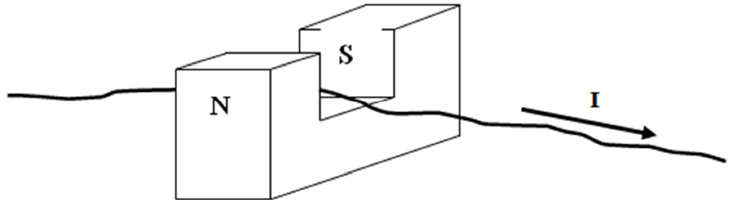• The direction of current is determined by how the wired is connected to the battery
• Observation: When the battery is switched on, the wire moves either upwards or downwards. Determine the correct direction using the right-hand palm rule before describing your observation of the investigation.
• For example, in the diagram above: the wire moves upwards when the battery is switched on.
• Quantitative analysis: the magnitude of the force experienced by the wire is dependent on:
• strength of magnetic field B produced by the horse-shoe magnet
• length of wire that is inside the magnetic field
• magnitude of current
• angle between current direction and the magnetic field lines (in your investigation, this is usually set to 90º to maximise the resultant force. Greater force leads to a more obvious wire movement)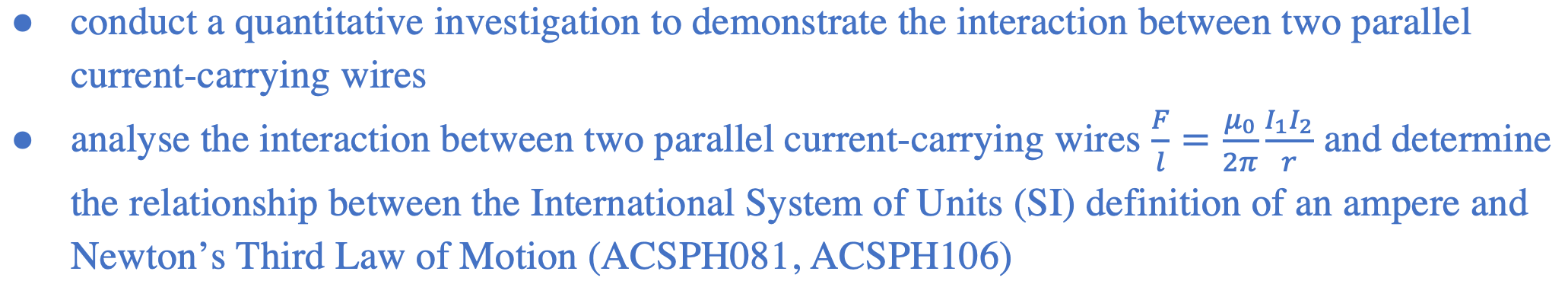### Force Between a Pair of Straight Parallel Current-carrying Conductors

•  A current carrying conductor generates its own magnetic field. The orientation of this magnetic field can be determined by using the right-hand rule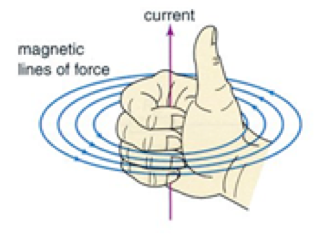• Two current carrying conductors produce magnetic fields which interact
• Magnetic fields whose field lines travel in the same rotational direction (either clockwise or anti-clockwise) lead to an attractive interaction
• Magnetic fields whose field lines travel in the opposite rotational direction (either clockwise or anti-clockwise) lead to a repulsive interactionQuantitative Investigation

• Consider two parallel conductors of infinite length, both containing current of 1 A, separated by a perpendicular distance of 1 m.
• The magnetic field strength produced by current in wire 2, experienced by wire 1 is• The magnetic force experienced by wire 1 therefore equals to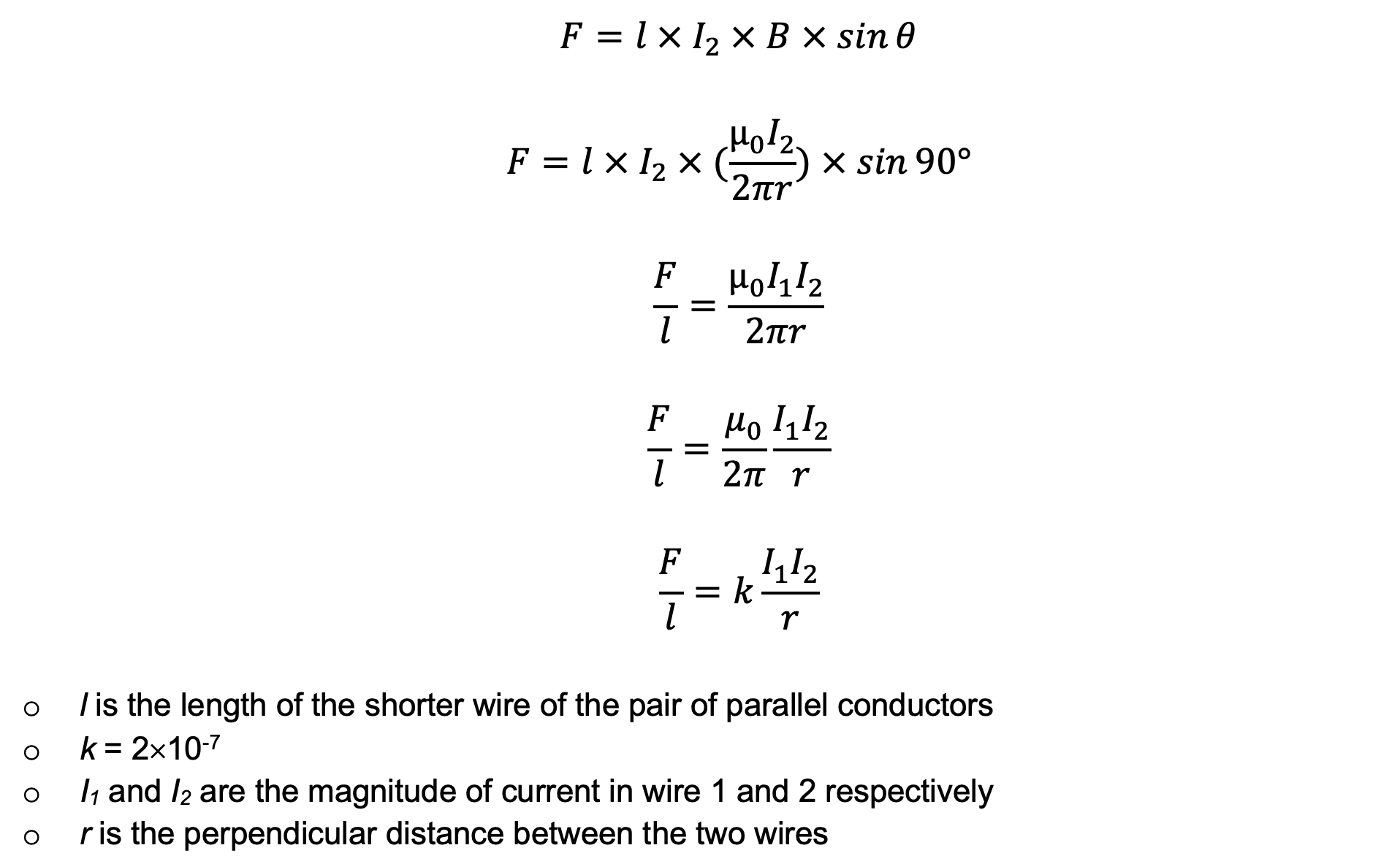• Thus, in this particular scenario: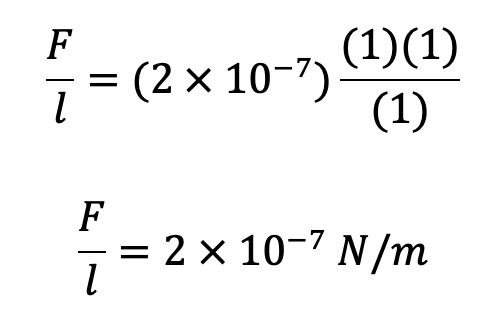• The magnitude and direction of this force can be investigated by resting one of the two parallel conductors on a scale. The second conductor is fixed directly above the conductor on the scale. Changes in scale reading will indicate the magnitude and direction of force
• Increase in reading à repulsive force à currents are travelling in opposite directions
• Decrease in reading à attractive force à currents are travelling in the same direction
• Many relationships can be investigated
• Current vs force
• Separation distance between conductors vs force
• Length of conductors

Practice Question 1

A 12.0 cm long wire is carrying a current of 3.0 A at 30° to a magnetic field of strength 0.9 T. Determine the magnetic magnitude and direction of the force on the wire. (2 marks)

Practice Question 2

Two conducting rails of negligible resistance are placed 80.0 cm apart. A conducting rod also with negligible resistance is placed across the rails. The mass of the rod is 800.0 g and there is negligible friction between the rod and rails. A 15.0 V battery is connected to the rails through a resistor. It was noticed that when a magnetic field of strength 0.4 T was applied at right angles to the inclined rails, the rod was sliding along the frictionless rails at a constant acceleration of 0.2 ms-2.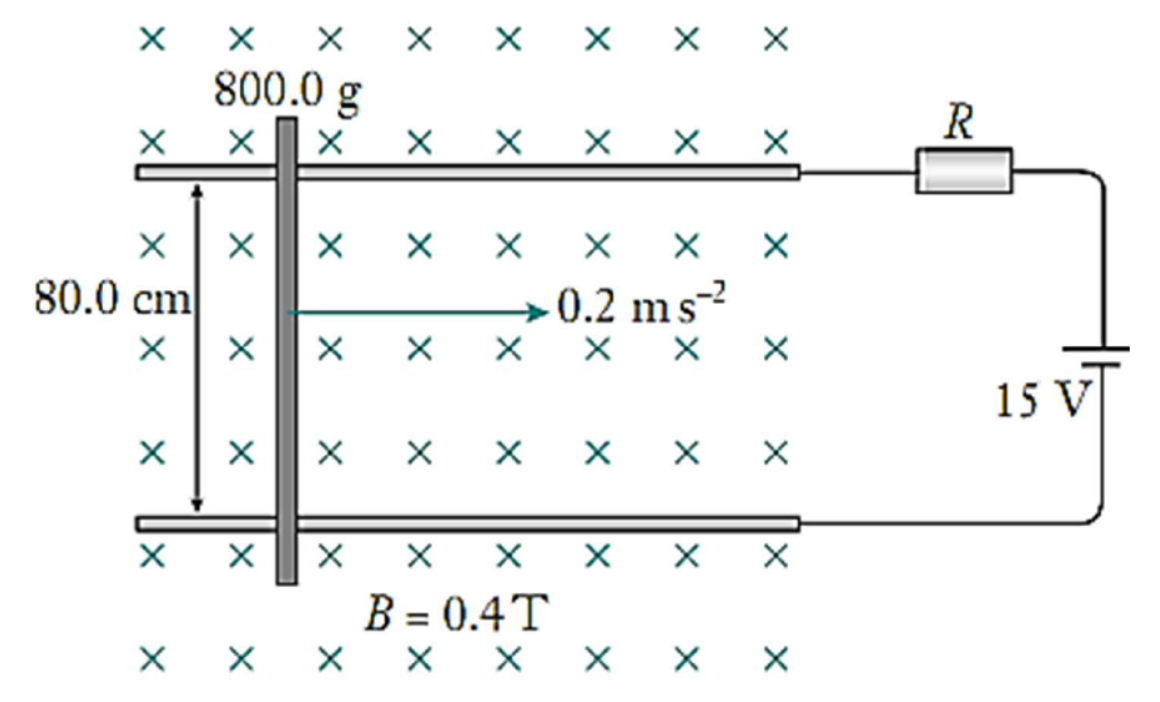(a) Calculate the net force acting on the rod (1 mark)

(b) Calculate the current in the circuit (2 marks)

(c) Calculate the resistance of the resistor (1 mark)

Practice Question 3

A wire of length 1.00 m and of mass 4.00 g is placed on top of a laboratory mass balance, which is calibrated to read in grams. A second wire, also 1.00 m long, is placed  m above is so that the two wires are parallel. A current of 12.0 A flows through each wire, in the direction shown in the diagram below.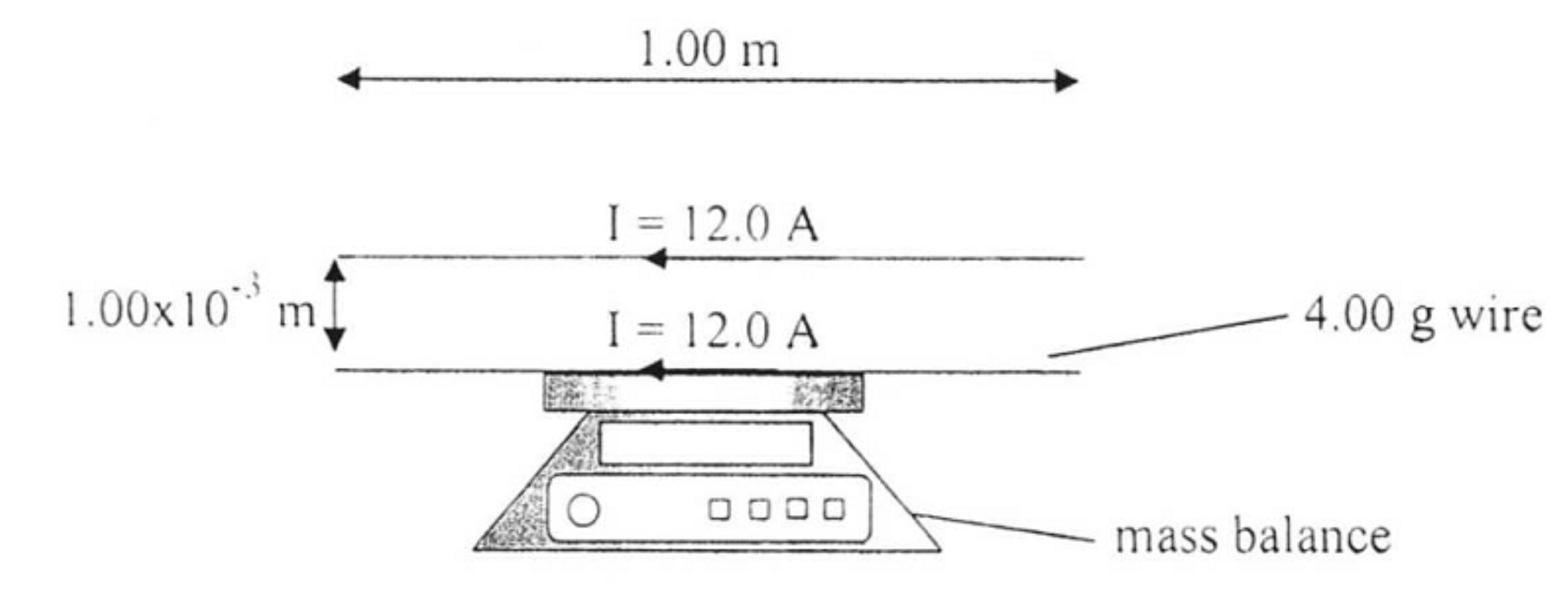(a) Calculate the force acting on the two parallel conductors. (2 marks)

(b) Calculate the reading on the mass balance. (2 marks)

Previous section: Charged Particle in Electric and Magnetic Fields

Next section: Electromagnetic Induction